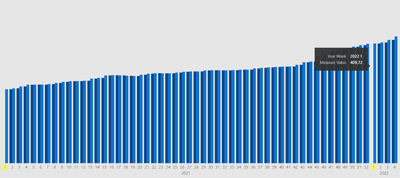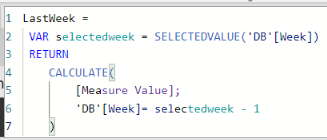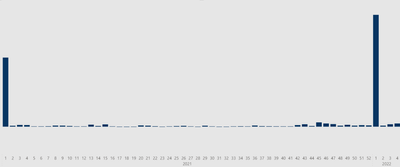cancel
Showing results for
Did you mean:Frequent Visitor

## Weekly variance calculations with year change

Hello,

I'm trying to calculate the weekly variance for the measures (So I've calculated two measures: "Measure" and "LastWeek") and I'm having problem with year change, therefore my "LastWeek" measures are not displayed in "2021 01" and "2022 01" columns (Only "Measure value" appears).• So for "LastWeek" column in week "2022 01" it should be calculated like this: "2022 01 - "2021 52".
• And for "2021 01" variance I'd like to see "0" variance.

Is it possible to update my DAX formula somehow to avoid these problems?LastWeek =
VAR selectedweek = SELECTEDVALUE('DB'[Week])
RETURN
CALCULATE(
[Measure Value];
'DB'[Week]= selectedweek - 1
)

Because of that, currently "Variance" Columns looks like this:1 ACCEPTED SOLUTIONCommunity Support

Are you trying to calculate current week's value minus previous week's value? If it is like this, please try following steps.

This is my test table:I suggest you trying to create new columns instead of measures.

``````Current_week_value =
var Current_date = MINX(FILTER('Table','Table'[Week] = EARLIER('Table'[Week]) && 'Table'[Year] = EARLIER('Table'[Year])),'Table'[Date])
return
IF('Table'[Date] = Current_date, CALCULATE(SUM('Table'[Value]),FILTER('Table','Table'[Year] = EARLIER('Table'[Year]) && 'Table'[Week] = EARLIER('Table'[Week])))
)

Previous_week_value =
var Current_date = MINX(FILTER('Table','Table'[Week] = EARLIER('Table'[Week]) && 'Table'[Year] = EARLIER('Table'[Year])),'Table'[Date])
return
IF('Table'[Date] = Current_date, CALCULATE(SUM('Table'[Value]),FILTER('Table','Table'[Week] = EARLIER('Table'[Week]) - 1 && 'Table'[Year] = EARLIER('Table'[Year])))
)

Last week variance =
var Final_week_2021 = CALCULATE(SUM('Table'[Value]), FILTER('Table','Table'[Year] = 2021 && 'Table'[Week] = MAX('Table'[Week])))
var Current_date = MINX(FILTER('Table','Table'[Week] = EARLIER('Table'[Week]) && 'Table'[Year] = EARLIER('Table'[Year])),'Table'[Date])
return
SWITCH(
TRUE(),
'Table'[Year] = 2021 && 'Table'[Week] = MIN('Table'[Week]) && 'Table'[Date] = Current_date, 0,
'Table'[Year] = 2022 && 'Table'[Week] = MIN('Table'[Week]) && 'Table'[Date] = Current_date, ABS([Current_week_value] - Final_week_2021),
'Table'[Date] = Current_date, ABS([Current_week_value]-[Previous_week_value])
)``````

Variance for 2021 01 is 0.Variance for 2022 01 is 2022 01 - 2021 53.I think this is the result you want:Best regards,

If this post helps, then please consider Accept it as the solution to help the other members find it more quickly.

3 REPLIES 3Community Support

Due to the inconsistency of our models,  in order to better understanding your demands and give the right solution, could you please share with me some screenshots of your data after hiding sensitive information.

Best regards,

If this post helps, then please consider Accept it as the solution to help the other members find it more quickly.Frequent Visitor

Thanks for the great and quick answer!
However, in my data model everything is pretty messed up, so your model wasn't counting the numbers in a right way, but, when I've placed only dates and values in a new workbook, your model worked perfectly fine.
After some more tutorials in YT, I've discovered the formula that works better with my messed data. But still, first column from 2021 01 is substracting the value with zero, as there are no more data from 2020 (This tutorial: https://www.youtube.com/watch?v=jclWnA7pEvY).Could you consult how should I add an exception in current formula for 2021 01 to show values as 0?
I've tried to take it from your formula but my attempts wasn't successful.
Basically I need to show zero value if Week is 1 and Year is 2021, else use VAR "MinWeeNumber".

Looks simple but I just can't use it right as I'm pretty new in DAX...TESTPW =
VAR CurrentWeek = SELECTEDVALUE('DB'[Week])
VAR CurrentYear = SELECTEDVALUE('DB'[PBIYear])
VAR MaxWeekNumber = CALCULATE(MAX('DB'[Week]); ALL('DB'))
VAR MinWeekNumber = SUMX(FILTER(ALL('DB');
IF( CurrentWeek = 1;
'DB'[Week] = MaxWeekNumber && 'DB'[PBIYear] = CurrentYear -1;
'DB'[Week] = CurrentWeek - 1 && 'DB'[PBIYear] = CurrentYear ));
[Measure Value])
RETURN
MinWeekNumberCommunity Support

Are you trying to calculate current week's value minus previous week's value? If it is like this, please try following steps.

This is my test table:I suggest you trying to create new columns instead of measures.

``````Current_week_value =
var Current_date = MINX(FILTER('Table','Table'[Week] = EARLIER('Table'[Week]) && 'Table'[Year] = EARLIER('Table'[Year])),'Table'[Date])
return
IF('Table'[Date] = Current_date, CALCULATE(SUM('Table'[Value]),FILTER('Table','Table'[Year] = EARLIER('Table'[Year]) && 'Table'[Week] = EARLIER('Table'[Week])))
)

Previous_week_value =
var Current_date = MINX(FILTER('Table','Table'[Week] = EARLIER('Table'[Week]) && 'Table'[Year] = EARLIER('Table'[Year])),'Table'[Date])
return
IF('Table'[Date] = Current_date, CALCULATE(SUM('Table'[Value]),FILTER('Table','Table'[Week] = EARLIER('Table'[Week]) - 1 && 'Table'[Year] = EARLIER('Table'[Year])))
)

Last week variance =
var Final_week_2021 = CALCULATE(SUM('Table'[Value]), FILTER('Table','Table'[Year] = 2021 && 'Table'[Week] = MAX('Table'[Week])))
var Current_date = MINX(FILTER('Table','Table'[Week] = EARLIER('Table'[Week]) && 'Table'[Year] = EARLIER('Table'[Year])),'Table'[Date])
return
SWITCH(
TRUE(),
'Table'[Year] = 2021 && 'Table'[Week] = MIN('Table'[Week]) && 'Table'[Date] = Current_date, 0,
'Table'[Year] = 2022 && 'Table'[Week] = MIN('Table'[Week]) && 'Table'[Date] = Current_date, ABS([Current_week_value] - Final_week_2021),
'Table'[Date] = Current_date, ABS([Current_week_value]-[Previous_week_value])
)``````

Variance for 2021 01 is 0.Variance for 2022 01 is 2022 01 - 2021 53.I think this is the result you want:Best regards,